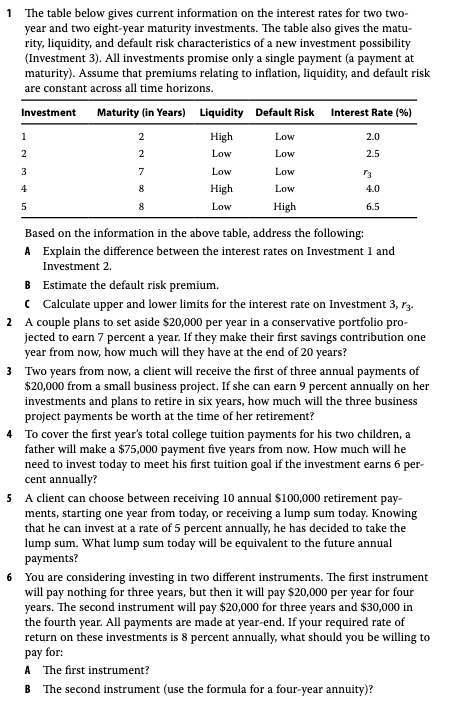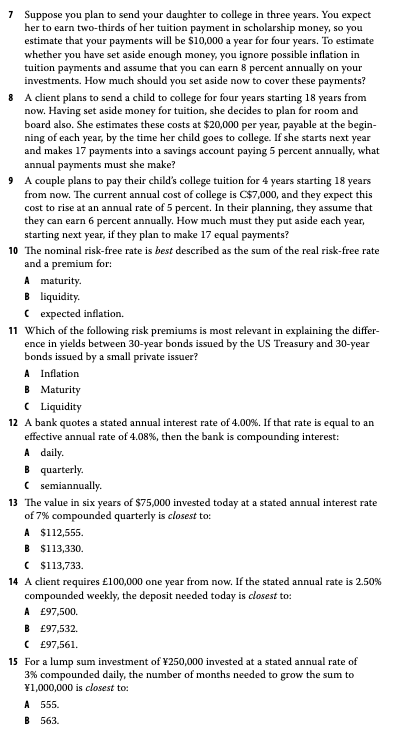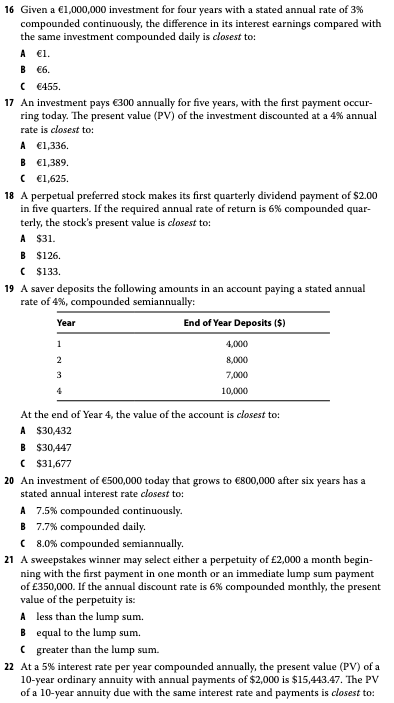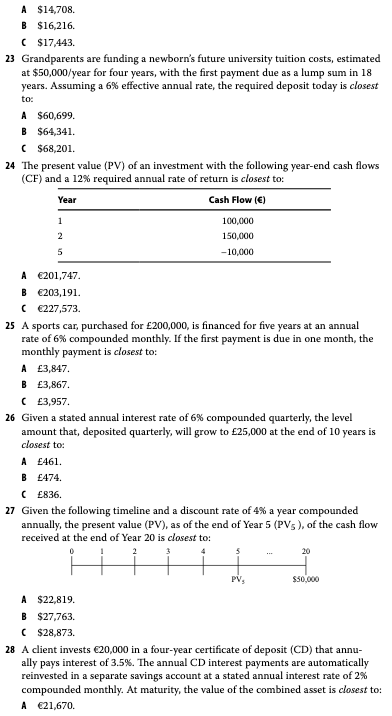In this post we will solve the end of the chapter practice problems from chapter 1 of the book.

#### Here are the first 6 problems and their solutions in R### Problem 1A

Investment 1 and 2 have the same maturity and default risks. However investment 2 has low liquidity and therefore investment 2 has a higher interest rate of 0.5% to compensate for the liquidity risk. This is the liquidity risk premium.

### Problem 1B

Investment 4 and 5 have the same maturity (8 years). We know from problem 1 the liquidity risk premiums is 0.5%. The interest rate difference between Investment 4 and 5, is 2.5%. Investment 5 has low liquidity and high default risk therefore it has a higher interest rate. Since we know 0.5% is the liquidity premium, we can attribute 2.5% - 0.5% = 2% as default risk premium.

### Problem 1C

Investment 2 and 3 has the same liquidity and default risk, but investment 3 has a higher maturity hence it should have atleast 2.5% as the lower bound. Investment 3 and 4 has low default risk. But investment 3 has a lower maturity and lower liquidity. We know the liquidity premium is 0.5%, but we don’t know the compensation for extra year of maturity. Therefore we could expect the interest rate for investment 3 to be between 2.5% and 4.5%.

### Problem 2

``````library(tidyverse)

savings <- 20000
interest <- 7/100
years <- 20
timeline <- rep(20000,years)

# Create a function to calculate the future value
# of payments

get_payment_fv <- function(x,y) {

payment <- (1 + interest) ^ (years - y) * x
return(payment)

}

payments <- map2_dbl(.x = timeline,.y = 1:years,
.f = get_payment_fv)

print(timeline)``````
``````##   20000 20000 20000 20000 20000 20000 20000 20000 20000 20000 20000
##  20000 20000 20000 20000 20000 20000 20000 20000 20000``````
``print(payments)``
``````##   72330.55 67598.65 63176.30 59043.27 55180.63 51570.68 48196.90
##   45043.83 42097.04 39343.03 36769.18 34363.72 32115.63 30014.61
##  28051.03 26215.92 24500.86 22898.00 21400.00 20000.00``````
``````cat("The couple will have", paste0("\$",round(sum(payments),2)),
"at the end of 20 years.")``````
``## The couple will have \$819909.85 at the end of 20 years.``

### Problem 3

``````cash_flow <- c(0, 0, 20000, 20000, 20000, 0, 0)
interest <- 9/100
years <- length(cash_flow)

payments <- map2_dbl(.x = cash_flow,
.y = 1:years,
.f = get_payment_fv)

cat("The client will have", paste0("\$",round(sum(payments),2)),
"at the end of 6 years.")``````
``## The client will have \$77894.21 at the end of 6 years.``

### Problem 4

``````college_fee <- 75000
interest <- 6/100
years <- 5

pv <- (1 + interest) ^ (-years) * college_fee

cat("The father will need to invest", paste0("\$",round(pv,2)),
"today to have \$75000 in 5 years at 6%")``````
``## The father will need to invest \$56044.36 today to have \$75000 in 5 years at 6%``

### Problem 5

``````interest <- 5/100
years <- 10

df <- tibble(year = 1:years,
cf = rep(100000,10)) %>%
mutate(pv = cf / (1 + interest)^ year)

print(df)``````
``````## # A tibble: 10 x 3
##     year     cf     pv
##    <int>  <dbl>  <dbl>
##  1     1 100000 95238.
##  2     2 100000 90703.
##  3     3 100000 86384.
##  4     4 100000 82270.
##  5     5 100000 78353.
##  6     6 100000 74622.
##  7     7 100000 71068.
##  8     8 100000 67684.
##  9     9 100000 64461.
## 10    10 100000 61391.``````
``````lump_sum <- sum(df\$pv)

cat("The client should expect", paste0("\$",round(lump_sum, 2)), "payment today.")``````
``## The client should expect \$772173.49 payment today.``

### Problem 6A

``````cf1 <- c(0,0,0,rep(20000,4))

interest <- 8/100

df <- tibble(year = 1:length(cf1),
cf = cf1) %>%
mutate(pv = cf / (1 + interest)^ year)

print(df)``````
``````## # A tibble: 7 x 3
##    year    cf     pv
##   <int> <dbl>  <dbl>
## 1     1     0     0
## 2     2     0     0
## 3     3     0     0
## 4     4 20000 14701.
## 5     5 20000 13612.
## 6     6 20000 12603.
## 7     7 20000 11670.``````
``````pv <- sum(df\$pv)

cat("We should be willing to pay",paste0("\$",round(pv,2)),"for the first investment")``````
``## We should be willing to pay \$52585.46 for the first investment``

### Problem 6B

``````cf2 <- c(rep(20000,3), 30000)
interest <- 8/100

df <- tibble(year = 1:length(cf2),
cf = cf2) %>%
mutate(pv = cf / (1 + interest)^ year)

cat("We should be willing to pay",paste0("\$",round(pv,2)),"for the second investment")``````
``## We should be willing to pay \$52585.46 for the second investment``

#### Problems 7 to 15 and their solution in R### Problem 7

``````cf <- c(rep(0,2), rep(10000,4))

interest <- 8/100

df <- tibble(year = 1:length(cf),
cf = cf) %>%
mutate(pv = cf / (1 + interest)^ year)

print(df)``````
``````## # A tibble: 6 x 3
##    year    cf    pv
##   <int> <dbl> <dbl>
## 1     1     0    0
## 2     2     0    0
## 3     3 10000 7938.
## 4     4 10000 7350.
## 5     5 10000 6806.
## 6     6 10000 6302.``````
``````pv <- sum(df\$pv)

cat("We should set aside", paste0("\$",round(pv,2)), "today to pay for the college tuition")``````
``## We should set aside \$28396.15 today to pay for the college tuition``

### Problem 8

``````# This is a two part problem
# First calculate the PV of
# Room and board required
# before college starts

cf1 <- rep(20000,4)
interest <- 5/100

df1 <- tibble(year = 1:length(cf1),
cf = cf1) %>%
mutate(pv = cf / (1 + interest)^ year)

fv <- sum(df1\$pv)

cat("The client will need", fv,
"before college starts.")``````
``## The client will need 70919.01 before college starts.``
``````# Now we need to calculate the
# payment needed to get this
# Future value

# We will use the FV value of annuity formula

# FV = annuity((1 + r)^N - 1 / r)
# we need to make 17 payments

annuity <- fv / (((1 + interest)^17 - 1) / 0.05)

cat("The parent will need to make", paste0("\$",round(annuity,2)), "to save for room and board")``````
``## The parent will need to make \$2744.5 to save for room and board``

### Problem 9

``````# First we need to calculate the
# Inflation adjusted cost of college
# in the future

inflation <- 0.05
tuition_today <- 7000
college_start_years <- 18

tuition_fv_year1 <- (1 + inflation) ^ college_start_years * tuition_today

cat("Tuition will be", paste0("C\$",round(tuition_fv_year1,2),
" for the first year, 18 years from now."))``````
``## Tuition will be C\$16846.33 for the first year, 18 years from now.``
``````# Now lets calculate the tuition payments for the next 3 years
# Year 0 is
df <- tibble(year = 1:4,
tuition = c(tuition_fv_year1, rep(0,3))) %>%
mutate(tuition = (1 + inflation)^(year - 1) * tuition_fv_year1)
print(df)``````
``````## # A tibble: 4 x 2
##    year tuition
##   <int>   <dbl>
## 1     1  16846.
## 2     2  17689.
## 3     3  18573.
## 4     4  19502.``````
``````# Now we need to PV these payments at
# beginning of year 17

interest <- 6/100 # Expected returns

df <- df %>%
mutate(pv = tuition / (1 + interest) ^ year)

fv_total_tuition <- sum(df\$pv)

# Now its an annuity problem as before

annuity <- fv_total_tuition / (((1 + interest)^17 - 1) / interest)
annuity``````
``##  2221.579``
``cat("The couple will need to save", paste0("\$",round(annuity,2)), "each year for the expected college tuition.")``
``## The couple will need to save \$2221.58 each year for the expected college tuition.``

### Problem 10

C. expected inflation.

C. Liquidity

### Problem 12

``(1 + 0.04/365)^365 - 1``
``##  0.04080849``

A. daily

### Problem 13

``(1+0.07 / 4) ^ (4 * 6) * 75000``
``##  113733.2``

A. \$113733

### Problem 14

``100000 / (1 + 0.025 / 52) ^ 52``
``##  97531.58``

B. 97532

### Problem 15

``````# Get the Effective annual rate
rate <- (1 + 0.03 / 365) ^ 365 - 1

# Get the months
# needed to quadruple the PV

log(1000000 / 250000) /  log(1 + rate) * 12``````
``##  554.5405``

A. 555

#### Problems 16 to 22 and their solution in R### Problem 16

``````# 3% continuously compounding
# for 4 years

continuous_compounding <- exp(0.03 * 4) * 1000000

daily_compounding <- (1 + 0.03 / 365) ^ (4 * 365) * 1000000

continuous_compounding - daily_compounding``````
``##  5.55994``

B. €6

### Problem 17

``````rate <- 4/100
pmt <- 300
years <- 5

pv <- (pmt / rate * (1 - 1/(1 + rate)^years)) * (1 + rate)

pv``````
``##  1388.969``

B. \$1389

### Problem 18

``````quarterly_div <- 2
yearly_div <- quarterly_div * 4

# PV a year from now

fv <- yearly_div / 0.06

# Current price

pv <- fv / (1 + 0.06)
pv``````
``##  125.7862``

B. \$126

### Problem 19

``````rate <- 4/100
ear <- (1 + rate / 2) ^ 2

df <- tibble(year = 1:4,
years_remaining = 4:1,
cf = c(4000, 8000, 7000, 10000)) %>%
mutate(fv = cf * ((1 + 0.02) ^ ((years_remaining - 1) * 2)))

df``````
``````## # A tibble: 4 x 4
##    year years_remaining    cf     fv
##   <int>           <int> <dbl>  <dbl>
## 1     1               4  4000  4505.
## 2     2               3  8000  8659.
## 3     3               2  7000  7283.
## 4     4               1 10000 10000``````
``````fv <- sum(df\$fv)

fv``````
``##  30446.91``

B. \$30447

### Problem 20

``````# continuously

cont <- exp(0.075 * 6) * 500000

# daily

d <- (1 + 0.07 / 365) ^ (6 * 365) * 500000

# Semiannually

s <- (1 + 0.08 / 2) ^ (6 * 2) * 500000

tibble(continuously = cont,
daily = d,
semiannually = s)``````
``````## # A tibble: 1 x 3
##   continuously   daily semiannually
##          <dbl>   <dbl>        <dbl>
## 1      784156. 760950.      800516.``````

C. 8.0% compounded semiannually

### Problem 21

``````perp_payment <- 2000 / (0.06 / 12)

perp_payment``````
``##  4e+05``

C. greater than the lump sum

### Problem 22

``````ordinary_annuity <- tibble(year = 1:10,
cf = 2000) %>%
mutate(pv = cf / (1 + 0.05) ^ year) %>%
summarise(s = sum(pv)) %>%
.[]

annuity_due <- ordinary_annuity * 1.05
annuity_due``````
``##  16215.64``

B. 16216

#### Problems 23 to 28 and their solution in R### Problem 23

``````rate <- 6/100
pmt <- 50000
years <- 4
# PV of ordinary annuity
# This is also the future value

fv <- (pmt / rate * (1 - 1/(1 + rate)^years))

# Calculate the PV

pv <- fv / (1 + rate) ^ 17
pv``````
``##  64340.85``

B. 64341

### Problem 24

``````rate <- 12/100

tibble(year = c(1,2,5),
cf = c(100000, 150000, -10000)) %>%
mutate(pv = cf / (1 + rate) ^ year) %>%
summarise(s = sum(pv)) %>%
.[]``````
``##  203190.5``

B. 203191

### Problem 25

``````rate <- 0.06/12
n <- 12 * 5

pv_factor <- (1 - (1/(1+rate) ^ n)) / rate

payment <- 200000 / pv_factor

payment``````
``##  3866.56``

B. 3866

### Problem 26

``````rate <- 0.06 / 4
n = 4 * 10

25000 / (((1 + rate) ^ n - 1) / rate)``````
``##  460.6775``

A. 460.68

``(50000 / (1 + 0.04)^20) * (1 + 0.04) ^ 5``
``##  27763.23``

B. \$27763

### Problem 28

• 21670
• 22890
• 22950
``````p <- 0.035 * 20000
p``````
``##  700``
``````rate <- 0.02/12
n <- 4 * 12

fv_payments <- tibble(years_remaining = 3:0,
cf = p) %>%
mutate(n = years_remaining * 12) %>%
mutate(fv = (1 + rate) ^ n * cf) %>%
summarise(s = sum(fv))

20000 + fv_payments``````
``````##          s
## 1 22885.92``````

B. 22890